# Basic Limit Laws

Listed here are a couple of basic limits and the standard limit laws which, when used in conjunction, can find most limits. They are listed for standard, two-sided limits, but they work for all forms of limits. However, note that if a limit is infinite, then the limit does not exist.

## Basic Limits

If c is a constant, then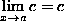.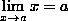.

## Limit Laws

If the limits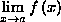and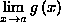both exist, then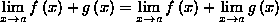.

### Subtraction Law

If the limitsandboth exist, then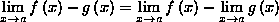.

### Constant Law

If c is a constant, and the limitexists, then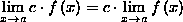.

### Multiplication Law

If the limitsandboth exist, then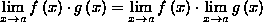.

### Division Law

If the limitsandboth exist, and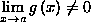, then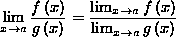.

### Power Law

If n is an integer, and the limitexists, then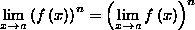.

### Root Law

If n is an integer, the limitexists, and that limit is positive if n is even, then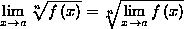.

### Squeeze Law

If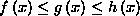for all x in an open interval that contains a, except possibly at a itself, and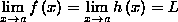, then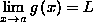.

### Composition Law

If f is continuous at b and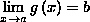, then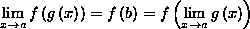.